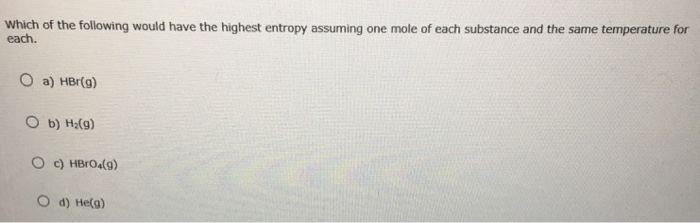# Problem: Which of the following would have the highest entropy assuming one mole of each substance and the same temperature for each. a) HBr(g) b) H2(g) c) HBrO4 d) He(g)

###### FREE Expert Solution

Entropy can be related to # atoms present in the molecule

97% (83 ratings)###### Problem Details

Which of the following would have the highest entropy assuming one mole of each substance and the same temperature for each.

a) HBr(g)

b) H2(g)

c) HBrO

d) He(g)